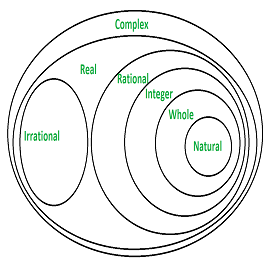Related Articles

# Show that 0.3333… = 0.3, can be expressed in the form of p/q

• Last Updated : 05 Aug, 2021

Ever since humans developed senses, they started keeping records of things. This led to the generation of the number system. Initially, there were only natural numbers in the number system. As the human brain developed more and more research was done and new things were invented and introduced. With the advent of time zero, negative integers, rational numbers, irrational numbers, real numbers, and complex numbers were invented.

The symbols for different types of numbers are as follows:

N ⇢ represents natural numbers.

W ⇢ represents whole numbers.

Z ⇢  represents integers.

Q ⇢ represents rational numbers.

P ⇢ represents irrational numbers.

R ⇢ represents real numbers.

z ⇢ represents a number in complex plane.

The following diagram shows the hierarchy of the number system,### Rational Numbers

The word ‘rational’ is derived from the word ‘ratio’. A number is said to be rational if it can be represented in the form of p/q, where p and q are integers and q ≠ 0. All rational numbers are real numbers. All positive integers, positive fractions, zero, negative integers, and negative fractions are part of the set of rational numbers. The set of rational numbers is denoted by Q.

Properties of rational numbers

1. Basic arithmetic can be performed, operations like addition, subtraction, multiplication, and division on rational numbers. The end result of these operations will result in rational numbers.
2. All rational numbers display commutative, associative and distributive properties for the operations of addition and multiplication.
3. The rational numbers have 0 and 1 as identity elements for the operation of addition and multiplication respectively.
4. For every rational number x there exist a rational number -x so that x+(-x)=0.
5. For every rational number x there exist a rational number 1/x such that x× (1/x)=1.

Decimal property of rational number

It is known that all rational numbers can be expressed in the form p/q where p and q are integers provided q ≠ 0. p divided q can result into an integer, terminating decimal, or repeating decimal. Let’s solve the given problem statement,

### Show that 0.3333… = 0 3, can be expressed in the form of rational number, i.e. p/q.

Now, let x = 0.33333…      -(1)

Multiplying (1) by 10 we get

10x = 3.33333…          -(2)

Now, subtracting (1) from (2) we get

9x = 3

⇒ x = 3/9

⇒ x = 1/3.

Hence, 0.33333 in rational form is 1/3.

### Similar Questions

Question 1: Express 0.40777777… in rational form.

Solution

Let x = 0.40777777…     -(1)

Multiplying (1) by 100 we get

100x = 40.777777…     -(2)

Multiplying (2) by 10 we get

1000x = 407.777777..    -(3)

Now, subtracting (1) from (2) we get

900x = 367

⇒ x = 367/900

Hence, 0.40777777… in rational form is 367/900.

Question 2: Express 1.0636363… in rational form.

Solution:

Let x = 1.0636363…     -(1)

Multiplying (1) by 10 we get

10x = 10.636363…     -(2)

Multiplying (2) by 100 we get

1000x = 1063.636363…  -(3)

Now, subtracting (2) from (3) we get

990x = 1053

⇒ x = 1053/990

Hence, 1.0636363… in rational form is 1053/990.

Attention reader! All those who say programming isn’t for kids, just haven’t met the right mentors yet. Join the  Demo Class for First Step to Coding Coursespecifically designed for students of class 8 to 12.

The students will get to learn more about the world of programming in these free classes which will definitely help them in making a wise career choice in the future.

My Personal Notes arrow_drop_up